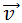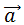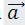# Collision of Two Particles

BeyondBelief96

## Homework Statement

A particle A moves along the line y = d (30 m) with a constant velocity(v= 3.0 m/s) directed parallel to the positive x axis (Fig. 4-40). A second particle B starts at the origin with zero speed and constant acceleration(a = 0.40 m/s2) at the same instant that particle A passes the y axis. What angle θ betweenand the positive y axis would result in a collision between these two particles?[/B]

## Homework Equations

Kinematic Equations

## The Attempt at a Solution

So I listed out all knowns and unknowns for both particle A & B as follows:

Particle A: Xi = 0, Xf = ?, Vix = 3 m/s, Vif = 3m/s, a = 0 t = ? and y = 30

Particle B: Xi = 0, Xf = ?, Vix = 0 m/s, Vif = ?, ax = asin(theta), t = ?
Yi = 0, Yf = 30, Viy = 0 m/s, Vif = ?, ay = acos(theta), t = ?

From there I used the kinematic equation d = vit + 1/2at^2 for particle A and B and got the following:

Particle A: x = 3t, y =30
Particle B: x = 1/2asin(theta)t^2, y = 1/2acos(theta)t^2

I set each of the equations equal to each other and tried to solve the system of equations for theta as follows:

3t = 1/2asin(theta)t^2 (Eq1)

30 = 1/2acos(theta)t^2 (Eq2)

3 = 1/2asin(theta)t so t = 6/asin(theta)

So I plugged t into Eq2:

30 = 1/2acos(theta)[6/asin(theta)]^2

from here im at a loss on how to solve for theta. To be honest, my algebra solving skills arent super spectacular, which is a bit sad haha. I was able to simplify this as:

30 = 1/2acos(theta)[36/a^2sin^2(theta)] == 5/3 = cot(theta)/asin(theta) .....aaaaaand this is where im lost.
[/B]

Homework Helper

## Homework Statement

A particle A moves along the line y = d (30 m) with a constant velocity View attachment 229683 (v= 3.0 m/s) directed parallel to the positive x axis (Fig. 4-40). A second particle B starts at the origin with zero speed and constant acceleration View attachment 229681 (a = 0.40 m/s2) at the same instant that particle A passes the y axis. What angle θ between View attachment 229682 and the positive y axis would result in a collision between these two particles?[/B]

## Homework Equations

Kinematic Equations

## The Attempt at a Solution

So I listed out all knowns and unknowns for both particle A & B as follows:

Particle A: Xi = 0, Xf = ?, Vix = 3 m/s, Vif = 3m/s, a = 0 t = ? and y = 30

Particle B: Xi = 0, Xf = ?, Vix = 0 m/s, Vif = ?, ax = asin(theta), t = ?
Yi = 0, Yf = 30, Viy = 0 m/s, Vif = ?, ay = acos(theta), t = ?

From there I used the kinematic equation d = vit + 1/2at^2 for particle A and B and got the following:

Particle A: x = 3t, y =30
Particle B: x = 1/2asin(theta)t^2, y = 1/2acos(theta)t^2

I set each of the equations equal to each other and tried to solve the system of equations for theta as follows:

3t = 1/2asin(theta)t^2 (Eq1)

30 = 1/2acos(theta)t^2 (Eq2)

3 = 1/2asin(theta)t so t = 6/asin(theta)

So I plugged t into Eq2:

30 = 1/2acos(theta)[6/asin(theta)]^2

from here im at a loss on how to solve for theta. To be honest, my algebra solving skills arent super spectacular, which is a bit sad haha. I was able to simplify this as:

30 = 1/2acos(theta)[36/a^2sin^2(theta)] == 5/3 = cot(theta)/asin(theta) .....aaaaaand this is where im lost.
[/B]
Here is a technique that you can use on a lot problems like this to eliminate angle ##\theta##.

##3t = \frac 1 2 a\,t^2 \sin(\theta)##
##30 = \frac 1 2 a\,t^2 \cos(\theta)##

##9t ^2= \frac 1 4 a^2t^4 \sin^2(\theta)##
##900 = \frac 1 4 a^2t^4 \cos^2(\theta)##
_____________________
##9t ^2 + 900 = \frac 1 4 a^2t^4##

In the last step, I just added the two equations. Now you have a quadratic equation to solve for ##t^2##.

BeyondBelief96
Here is a technique that you can use on a lot problems like this to eliminate angle ##\theta##.

##3t = \frac 1 2 a\,t^2 \sin(\theta)##
##30 = \frac 1 2 a\,t^2 \cos(\theta)##

##9t ^2= \frac 1 4 a^2t^4 \sin^2(\theta)##
##900 = \frac 1 4 a^2t^4 \cos^2(\theta)##
_____________________
##9t ^2 + 900 = \frac 1 4 a^2t^4##

In the last step, I just added the two equations. Now you have a quadratic equation to solve for ##t^2##.

NEW
oh okay, that makes sense! So once I've solved for t^2 just square root it for t, and then plug it into one of my original equations then solve for theta?

Homework Helper
NEW
oh okay, that makes sense! So once I've solved for t^2 just square root it for t, and then plug it into one of my original equations then solve for theta?
Right.